## INTERLEAVED INVERTING CHARGE PUMPA new topology for low noise negative voltage supplies

From Jon Kraft, Steve Knoth

Related Vendorsless

Analog Devices offers a wide variety of solutions for producing low noise power. Most of these solutions are designed to produce positive voltage rails, with fewer dedicated ICs for generating negative voltages. This can be particularly limiting when the negative voltage needs to power low noise devices, such as RF amplifiers, switches, and data converters.

Noise must be minimized in precision instrumentation or radio frequency (RF) circuits, but reducing noise comes with a number of challenges due to the nature of these systems. For instance, these systems must often operate over a wide input voltage while meeting strict electromagnetic interference (EMI) and electromagnetic compatibility (EMC) requirements. Furthermore, systems are crowded with electronics, making them space-constrained and heat sensitive. The increasing complexity of integrated circuits (ICs) has led to an increase in the number of power supply voltage rails that these systems require. Generating all these rails, meeting the above requirements, and keeping the entire system low noise can be daunting.

In this article, a new method to generate this low noise negative rail from a positive supply is introduced. It starts with a general understanding of how negative rails are typically generated and where they are used. Then we discuss the standard inverting charge pump before introducing an interleaved inverting charge pump (IICP) topology. A short derivation of the input and output voltage ripple for the IICP emphasizes its unique advantages for low noise systems.

### Traditional negative voltage generation methods

To create a negative voltage, one of two methods is commonly employed: use an inductive switching regulator or use a charge pump. Inductive switchers use an inductor or transformer to generate the negative voltage. Examples of these magnetic converter topologies are: inverting buck, inverting buck-boost, and Ćuk. Each of these has its own set of advantages and disadvantages regarding solution size, cost, efficiency, noise generation, and control loop complexity.1), 2) In general, the magnetics-based converters are best suited when higher output currents are required (> 100 mA).

For applications requiring less than 100 mA of output current, charge pump positive-to-negative (inverting) dc-to-dc converters can be very small and feature low EMI because no inductors or control loops are required. They simply require moving charge between capacitors via switches—supplying the resulting charge to the output.

Because charge pumps use no magnetics (inductors or transformers), they typically feature lower EMI than inductive switching topologies. Inductors tend to be much larger than capacitors, and unshielded inductors act like antennas by broadcasting radiated emissions. In contrast, the capacitors used in a charge pump do not produce any more EMI than a typical digital output. They can be easily routed in short traces to reduce antenna area and capacitive coupling, resulting in lower EMI.

Table 1 compares inductor-based switching regulator and switched capacitor inverting topologies.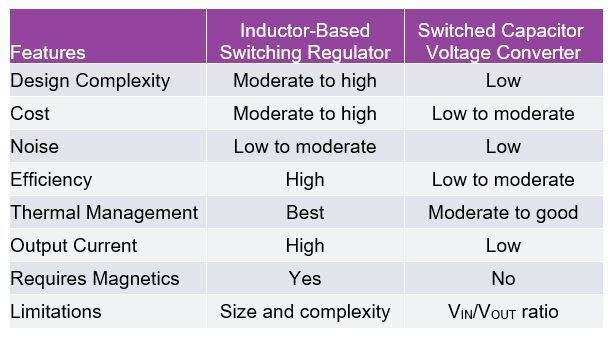Table 1. Comparison of magnetic and inverting charge pumps. (Source: Analog Devices)

The configuration of the traditional inverting charge pump is shown in Figure 1.

The output impedance, ROUT, of the charge pump is defined as the equivalent resistance of the charge pump mechanism from input to output. It is found by measuring the input to output voltage difference and dividing by the load current (see Equation 1).

Alternatively, the equivalent output resistance can be calculated as a function of switching frequency, switch resistance, and flyback capacitor size—generally simplified as is shown in Equation 2.

Each of the four switches operates at the same frequency, fOSC, and they are on for one half of the switching period, T, where T = 1/fOSC. Operation can be separated into two phases based on the two halves of the switching period, as shown in Figure 2.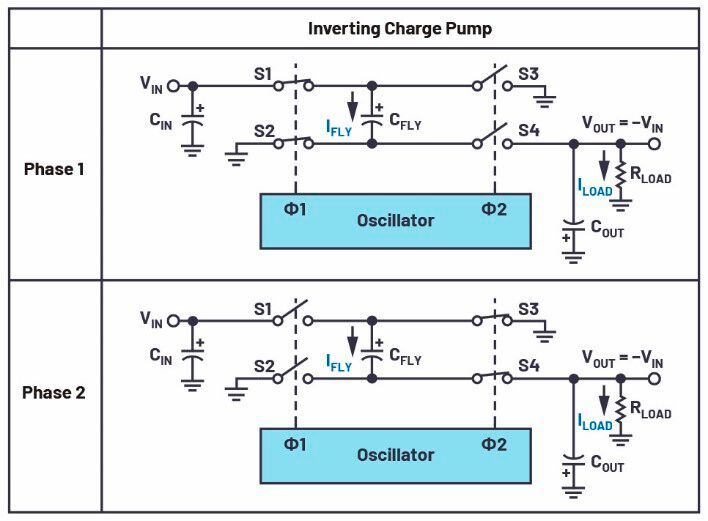Figure 2. Inverting charge pump during each phase of operation. (Source: Analog Devices)

Figure 3 gives the voltages and currents for each phase of the charge pump’s operation. In Phase 1, S1 and S2 are closed and S3 and S4 are open. This charges the flying capacitor (CFLY) to a voltage of +VIN. In Phase 2, the energy from CFLY is discharged into the output by opening S1 and S2 and closing S3 and S4. The two distinct phases of operation means that discontinuous current flows into CFLY from VIN, and discontinuous current flows out of CFLY into COUT. This leads to voltage ripple on CIN and COUT, which can be calculated as shown in Equation 3, 4, and 5.

Equation 4 and Equation 5 illustrate that, for a standard inverting charge pump, the voltage ripple is a function of switching frequency and input (or output) capacitance. Higher frequency and higher capacitance reduce this ripple in a 1:1 relationship. However, there are practical impediments to increasing frequency: namely increasing current consumption of the chip, which decreases efficiency.

### Subscribe to the newsletter now

#### Don't Miss out on Our Best Content

Unfold for details of your consent

Similarly, cost and PCB area often restrict the maximum input and output capacitance of an inverting charge pump. Also note that the flyback capacitor plays no role in the charge pump’s voltage ripple.

To reduce ripple, input and output filters could be constructed around the charge pump, but this again increases complexity and the charge pump’s output resistance. However, these issues can be addressed with a novel improvement to the standard inverting charge pump inverter: an interleaved inverting charge pump (IICP).

### Interleaved inverting charge pump (IICP)

Phase interleaving is widely used in inductive switching regulators (that is, polyphase operation) to reduce output voltage ripple.3) A 2-phase buck converter interleaved at exactly 50 % duty cycle produces, in theory, 0 mV of output voltage ripple. Of course, the duty cycle of a regulated buck converter changes with input and output voltage, so the 50 % case is only realized when VIN = 2 VOUT. Charge pumps usually operate at exactly 50 % duty cycle, so an interleaved charge pump inverter is interesting to consider.

Interleaving charge pumps are sometimes used within ICs when a very low current negative rail is required on the die, but right now there is no commercially available dedicated IICP inverting dc-to-dc converter. The construction of an IICP requires two charge pumps and two flying capacitors. The second charge pump operates the switches 180° out of phase with the first charge pump. Let’s look at the setup and the output ripple of an IICP and highlight how to optimize its performance. The setup is shown in Figure 4 with the timing diagram in Figure 5.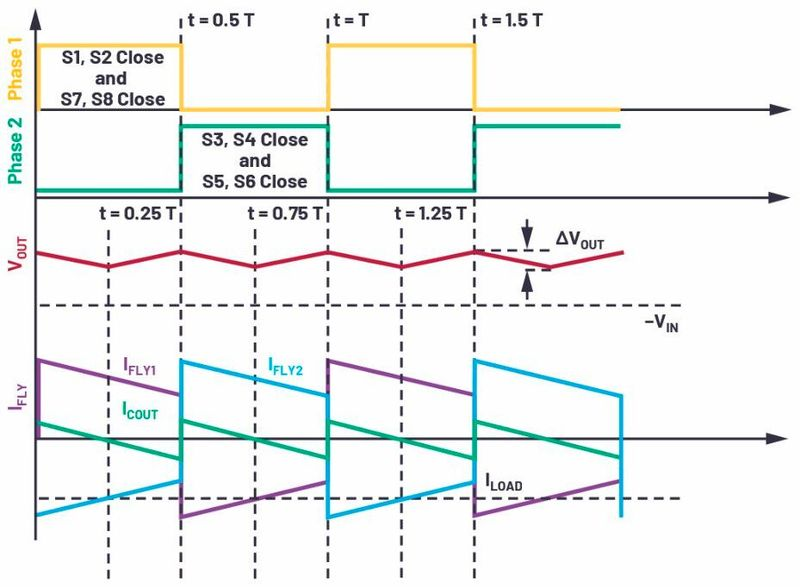Figure 5. Timing diagram for interleaved inverting charge pump. (Source: Analog Devices)

In each phase of the oscillator, one of the flying capacitors is connected to VIN and the other is connected to VOUT. At first glance, one might think that the addition of the second capacitor would only reduce the voltage ripple by half. However, this is an inaccurate oversimplification. In fact, the input and output voltage ripple can be far less than a standard inverter, because a capacitor is always charging from the input and discharging to the output. This can be better understood from the derivation of the IICP’s output voltage ripple.

### IICP output voltage ripple derivation

Since the IICP always has one of the flying capacitors supplying current to the output, its output stage can be simplified, as shown in Figure 6.

Furthermore, the IICP’s output resistance, as defined in Equation 1, can be approximated by Equation 6, and 7.

The output voltage ripple, ∆VOUT, is dVOUT and VCFLY(t) is the voltage difference across CFLY. We can make the reasonable assumption that output voltage ripple is small relative to the flying capacitor voltage ripple. Then to calculate ∆VOUT, we need an understanding of VCFLY(t). From Figure 6, note that IFLY is equal to the current through the two on switches. And each of those switches has a resistance of RON. Therefore see Equation 8.

To solve this differential equation for VCFLY(t), at least one initial condition must be known. This condition can be found via inspection of the timing graphs in Figure 5. Note that from t = 0 to t = T/4, both CFLY capacitors contribute current to ILOAD and charge COUT. Then, from t = T/4 to t = T/2, CFLY and COUT contribute to the output load current. So, right at t = T/4 (and similarly t = 3/4 T), the contribution to ILOAD from COUT is exactly 0. Therefore, at this moment, ILOAD is equal to IFLY, and the voltage of VCFLY is given by Equation 9.

Using Equation 8 and Equation 9, we can differentially solve for VCFLY(t) as is shown in Equation 10.

To find the delta in VCFLY for Equation 7, take two points (for example, t = 0 and t = T/4), and solve Equation 10 for each of those points. The result simplifies to as is shown in Equation 11.

Combining Equation 11 and Equation 7, and solving for ∆VOUT gives Equation 12.

The impact of Equation 12 may not be initially obvious. It may help to first simplify it by considering the case of an ideal switch (RON = 0 Ω). Doing so brings the second term to nearly zero, leaving only the first term. That first term is very similar to the standard inverting charge pump ripple (Equation 4), but the dual flying capacitors of the IICP increase the denominator by 2×. Twice the charge pumps yields half the ripple. This result is consistent with intuition.

However, the important part of Equation 12 lies in the second half. Note the minus sign for the second term, meaning that this portion reduces the output voltage ripple. Focus on the switch resistance (RON) and the flying capacitor (CFLY). In a standard inverting charge pump, these terms play no role in reducing the output voltage ripple. But in an IICP, the switch resistance acts to smooth out the charge and discharge current. The dual flying capacitors allow this charge/discharge action to happen uninterrupted.

### Output voltage ripple confirmation

We can use circuit simulation to check the accuracy of Equation 12 and the validity of the assumptions used to derive it. This is easily accomplished using LTspice. The schematic for this simulation is shown in Figure 7.
A comparison was performed for a variety of conditions, with a summary of the results in Table 2.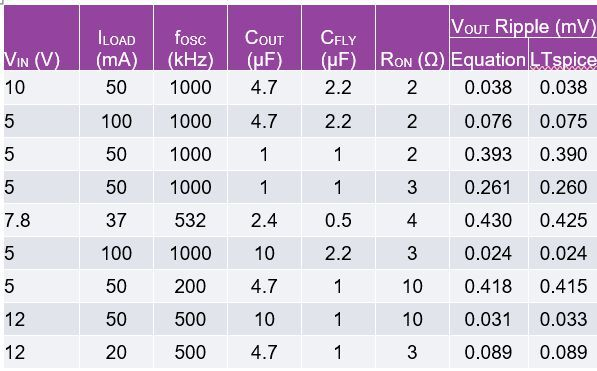Table 2. Comparison of theoretical vs. LTspice simulation results for various configurations. (Source: Analog Devices)

Table 2 shows that Equation 12 closely matches simulation, validating the assumptions made in simplifying the equations. Now we can use that equation to make trade-offs in the IICP implementation.

It’s also instructive to compare the voltage ripple between an IICP and a standard charge pump. In Part 2 of this series, we will show bench test data of these differences. But for now, our LTspice model in Figure 8 can illustrate the difference in output voltage ripple.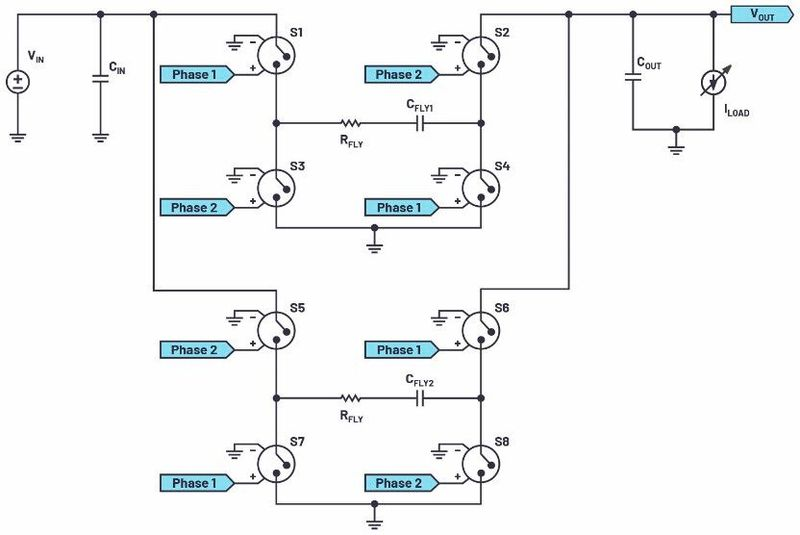Figure 7. Interleaved inverting charge pump in LTspice. (Source: Analog Devices)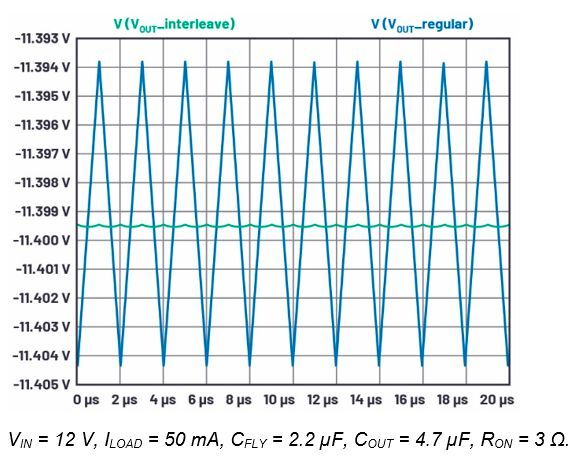Figure 8. Output voltage ripple of an IICP vs. a regular charge pump. (Source: Analog Devices)

### Optimization of IICP topology

Having derived the IICP equations and proved their validity, there are two primary conclusions: For the IICP, the switch resistance (RON) reduces both input and output voltage ripple, a desired result. In contrast, in a standard inverting charge pump, the switch resistance is entirely undesirable, as it increases the ROUT of the charge pump and provides no ripple voltage reduction. In fact, we could further augment the switch resistance by placing a resistor in series with the flyback capacitor. This gives us a knob to reduce input and output voltage ripple at the expense of increased charge pump resistance.

Secondly, the value of the flying capacitors, and their ratio with COUT, can be optimized to further optimize the ripple. For example, a large output capacitor value may be difficult to find in a small package, and subject to a significant capacitance derating at higher voltages. But by reducing COUT, and then increasing CFLY, the same output voltage ripple can be obtained for more attainable values of capacitance. For example, instead of CFLY = 1 µF and COUT = 10 µF, if they were all set to 2.2 µF, then nearly the same output voltage ripple is attained. 2.2 µF/25 V capacitors are more readily available in small packages than 10 µF/25 V capacitors.

### Conclusion

This article covered the general concepts behind an IICP topology, including input/output voltage ripple calculations. The derivation of the equations governing input/output ripple yields important insights into how to optimize the performance of an IICP solution.

### References

1) Jaino Parasseril. “How to Produce Negative Output Voltages from Positive Inputs Using a µModule Step-Down Regulator.” Linear Technology.
2) Kevin Scott and Jesus Rosales. “Differences Between the Ćuk Converter and the Inverting Charge Pump Converter.” Analog Devices, Inc.
3) Majing Xie. “High Power, Single Inductor, Surface-Mount Buck-Boost µModule Regulators Handle 36 VIN, 10 A Loads.” Linear Technology, March 2008.